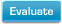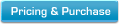New Features in Maple 15: Optimization Package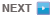Polynomial Arithmetic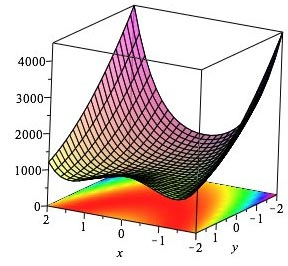The Optimization package provides many techniques for finding the minimum or maximum of an objective function subject to constraints. It solves linear programs, quadratic programs, nonlinear programs, and both linear and nonlinear least-squares problems, whether constrained or unconstrained. Variables may be continuous, integer or binary.

A new sparse interior-point method has been implemented for linear programs. The new algorithm is much more efficient than the existing, simplex-based active-set method for large, sparse problems and takes advantage of the sparse matrix package UMFPACK. Maple automatically determines the most efficient method to use based on the linear programming problem, or you can specify the method you want to use.

Here are some examples of the new interior-point method in action: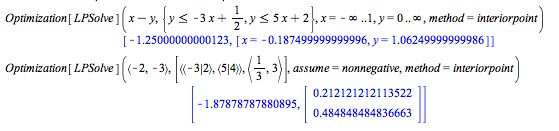The following plot shows a comparison between the two methods on a set of standard Netlib benchmark problems: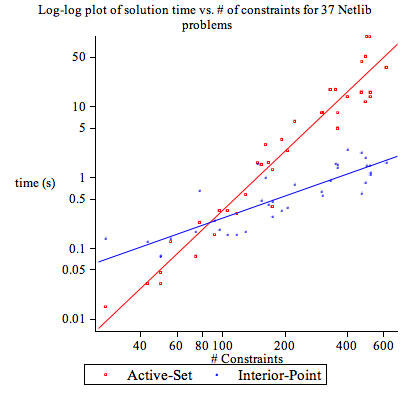Polynomial Arithmetic